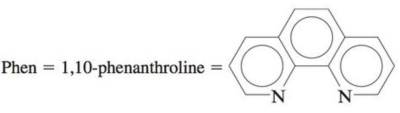# The complex ion Ru(phen) 3 2+ has been used as a probe for the structure of DNA. (Phen is a bidentate ligand.) a . What type of isomerism is found in Ru(phen) 3 2+ ? b . Ru(phen) 3 2+ is diamagnetic (as are all complex ions of Ru 2+ ). Draw the crystal field diagram for the d orbitals in this complex ion.### Chemistry: An Atoms First Approach

2nd Edition
Steven S. Zumdahl + 1 other
Publisher: Cengage Learning
ISBN: 9781305079243

#### Solutions

Chapter
Section### Chemistry: An Atoms First Approach

2nd Edition
Steven S. Zumdahl + 1 other
Publisher: Cengage Learning
ISBN: 9781305079243
Chapter 20, Problem 80AE
Textbook Problem
39 views

## The complex ion Ru(phen)32+ has been used as a probe for the structure of DNA. (Phen is a bidentate ligand.)a. What type of isomerism is found in Ru(phen)32+?b. Ru(phen)32+ is diamagnetic (as are all complex ions of Ru2+). Draw the crystal field diagram for the d orbitals in this complex ion.(a)

Interpretation Introduction

Interpretation: The type of isomerism found in Ru(phen)32+ and the crystal field diagram for the d orbitals in this complex ion is to be stated.

Concept introduction: The electrons in the d orbital of a transition metal split into high and low energy orbitals when ligands are attached to it. The energy difference between these two levels depends upon the properties of both metal and the ligands. If the ligand is strong, then splitting will be high and the complex will be low spin. If the ligand is weak, then splitting will be less and the complex will be high spin.

To determine: The type of isomerism found in Ru(phen)32+ .

### Explanation of Solution

Explanation

In Ru(phen)32+ , ruthenium is attached to three 1 , 10 -phenanthroline ligands. It exhibits optical isomerism since its mirror image is non super imposable

(b)

Interpretation Introduction

Interpretation: The type of isomerism found in Ru(phen)32+ and the crystal field diagram for the d orbitals in this complex ion is to be stated.

Concept introduction: The electrons in the d orbital of a transition metal split into high and low energy orbitals when ligands are attached to it. The energy difference between these two levels depends upon the properties of both metal and the ligands. If the ligand is strong, then splitting will be high and the complex will be low spin. If the ligand is weak, then splitting will be less and the complex will be high spin.

To determine: The crystal field diagram for the d orbitals in the Ru(phen)32+ complex.

### Still sussing out bartleby?

Check out a sample textbook solution.

See a sample solution

#### The Solution to Your Study Problems

Bartleby provides explanations to thousands of textbook problems written by our experts, many with advanced degrees!

Get Started

Find more solutions based on key concepts
Efforts in all of the following realms are necessary for weight change a. eating patterns, physical activity an...

Nutrition: Concepts and Controversies - Standalone book (MindTap Course List)

Calculate the mass in grams of 100.0mL of chloroform (d=1.49g/mL).

Chemistry for Today: General, Organic, and Biochemistry

A uniform solid sphere of radius r is placed on the inside surface of a hemispherical bowl with radius R. The s...

Physics for Scientists and Engineers, Technology Update (No access codes included)